## ABX Statistics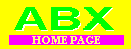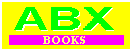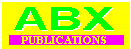ABX Company Publication P9 ABX Statistics -by David Carlstrom
 In the evaluation of ABX Double Blind Test results, statistics are used to get as much information as possible from each test. The statistical methods reviewed here are by no means the only ones available for use with an ABX Double Blind Comparator. They are however the methods that we at the ABX Company have found useful in ABX Double Blind Comparisons. Experienced researchers may utilize methods beyond the scope of this paper. One traditional approach to audibility thresholds defined the threshold point as midway between all correct (100%) and guessing (50%). Thus less than 75% correct indicated the effect was not detected by the listeners. With statistical evaluation, ABX Double Blind Comparisons can show audible differences with as little as 51% correct, provided the experiment is carefully designed. The statistics to bring this high level of resolution are elementary. The discussion to follow can serve both as an explanation for those new to statistics or as a reminder to statisticians who don't use these simple methods regularly. WHAT IS BEING TESTED ? Although novel experiments may be designed to utilize the Double Blind features of the ABX Comparator System, it was designed initially for comparisons of audio components. In this application, the ABX Company has adopted the following definitions:
 EXPERIMENTOR The person designing the experiment and doing the testing. LISTENER(S) The person(s) doing the listening. SUBJECT The audio equipment or audio parameter being investigated; that is the device under test (DUT) or parameter under test (PUT)
 It is important not to confuse SUBJECT and LISTENER. Listeners thinking their ears are on trial may be intimidated and thereby not do their best. This caution is worth explaining at the beginning of each test. Since the randomization of the ABX Comparator is internal, the EXPERIMENTER and the LISTENER may be the same person. With the ABX Comparator Double Blind tests may be done by a single person working alone. MISSING A FEW Listeners don't have to be correct on each and every response to show that the effect being tested is detectable. When some responses are incorrect, the science of statistics is called upon to show the responses do relate to the effect being tested. The statistics are basic, so basic that they are rarely explained in practical terms in statistics texts. WHAT IS p ? Statistics is a science of probabilities. There are no absolute tests of absolute proof. The result of a statistical analysis is also a probability. Whether a result is random or not random is stated statistically as the likelihood of the result being random. A result's randomness is determined by comparing the experimental result with results that have been theoretically studied. These reference points are called "distributions". To test if an ABX Double Blind score is random or a significant event, the score would be compared with a known distribution. Tossing a single coin repeatedly is statistically identical to the listeners score in an ABX Double Blind Comparison. The coin is random; its distribution is well known. In a very large number of tosses the head comes up half the time. If the listeners just guessed in an ABX Comparison, the score would likewise be 50%. If the score is above 50%, it may be random or not. Which depends on the number of trials run. Generally the evidence that the score was not like random guessing is stronger the more trials are run, although advanced researchers may be able to calculate an optimum number of trials in certain circumstances. We at the ABX Company have found sixteen trials to be fairly sensitive without an undue burden on the listeners. If a coin is tossed sixteen times, there won't always be exactly 8 heads. Five to eleven heads may occur quite frequently in each group of sixteen tosses. If twelve heads occur in sixteen tosses, the coin may be out of balance. The dividing line is between eleven and twelve because the coin toss distribution has been compared to other known distributions. The closest ones are the Chi-Squared Distribution and the Binomial Distribution. The Chi- Squared Test is easy to use by a simple calculation and a table of Chi-Squared valued and the corresponding probability values, but is approximate for small scores. The Binomial Distribution Test is exact but tables are not readily available for large numbers. The ABX Comparator User's Manual includes an exact Binomial Distribution Table for up to 40 trials. The ABX Company has prepared a Binomial Table for up to 600 trials. It is a 2 inch thick printout. [This was written in 1982.] USING THE BINOMIAL TABLE The table is easy to use. If, for example, the experiment's result was 20 X's out of 30 correctly identified, 20 is found across the top of the page and 30 at the side. The number at the row column intersection is the probability this score could have occurred in a coin toss or guessing situation. For 20/30, the probability listed in the table is 0.049. This means that in 100 random fair coin toss experiments consisting of 30 unknowns, a score of 20/30 or better would be expected to happen almost five times, but no more. Thus 20/30 is a fairly rare event. In a scientific report this would be stated: 67% correct (p=0.049). In rigorous scientific experiments the value of p is chosen in advance as a goal. The most common p value thus set is 0.05. Lower values are sometimes sought and achieved, but higher values are almost never tolerated. It would be unwise to report an audibility result if p is not below 0.05. USING CHI-SQUARED In the same 20 X's correct out of 30 X's example, a value of Chi-Squared is calculated by the formula below. The probability of the Chi-Squared value is determined from the Chi-Squared Table. In the case of an ABX Double Blind Comparison, the Chi-Squared value is: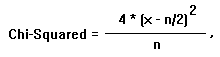where, x = number of X's correct, and n = number of X's attempted.
 In the example,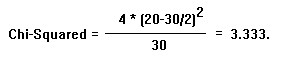In the Chi-Squared Table 3.333 lies above 3.170 and below 3.841. The table gives the probability of 3.170 as less than 0.075, so this is the best we can quote from this table. The advantage of the Exact Binomial Test is clear as it interprets this test as significant. We recommend the Binomial Table be used whenever possible. EXPERIMENTAL DESIGN The ABX Double Blind Comparison is set up as much as possible like the Double Blind Tests used by pharmaceutical houses to prove new medicines are effective. The ABX Comparator can of course be used in other experimental designs. In the scientific method an experiment is designed in advance and run only when the design is complete. The design sets what is to be tested by specifying a hypothesis which the experiment tests. In an ABX Double Blind Comparison both the hypothesis and its opposite are important:
 HYPOTHESIS: The difference between Component A and Component B can be heard. NULL HYPOTHESIS: A sounds the same as B.
 In the ABX Double Blind Comparison the goal is to statistically disprove the null hypothesis to confirm the hypothesis. When an individual ABX Comparision is completed, the responses are checked against the key in the ABX Comparator in ANSWER mode. The number of correct responses is compared to the number of response attempts to give a score such as the example of 20 correct out of 30 attempts, which we have written briefly as a fraction or a percentage: 20 / 30 = 67%. The score is then compared with the probability table from which the probability that it is a random score is determined. Thus the result is stated: 20 / 30 = 67% (p = 0.049). This literally means that the score (20/30) is probably not random except for a 0.049 chance that it is random. Thus the result of the experiment is that the null hypothesis is not true except for a 0.049 chance it is true, or in audiophile terms, A sound different from B except for a 4.9% chance that they are the same. Note that no matter what score is achieved, A = B cannot be proven. That is the ABX Double Blind Comparison can never be used to prove two audio components sound the same. The notion that ABX can prove components sound the same is a common misconception about ABX. A second common misconception about ABX is the claim that an ABX test result is not a preference: it doesn't tell which audio component sounds better. While literally true, if an ABX test confirms a difference is heard, selecting one's preference is easy and completely justified. If the score had been random, 19 / 30 = 63% (p = 0.1), all that could be reported is the experimentor failed to disprove the null hypothesis. No further conclusion could be reached about the similarity or difference of component A and component B from this Double Blind experiment. Of course a near miss score like 19 / 30 may tempt the experimenter to attempt more trials in the hope of the new score and the combined score being significant.

## Chi-Squared TableSend questions or comments on the ABX Web Page to David Carlstrom.